# Let C be the closed, piecewise smooth curve formed by traveling in straight lines between the points (−4, 1), (−4, −2),...

Let C be the closed, piecewise smooth curve formed by traveling in straight lines between the points (−4, 1), (−4, −2), (2, −1), (2, 6), and back to (−4, 1), in that order. Use Green's theorem to evaluate the following integral. C (2xy) dx + (xy^2) dy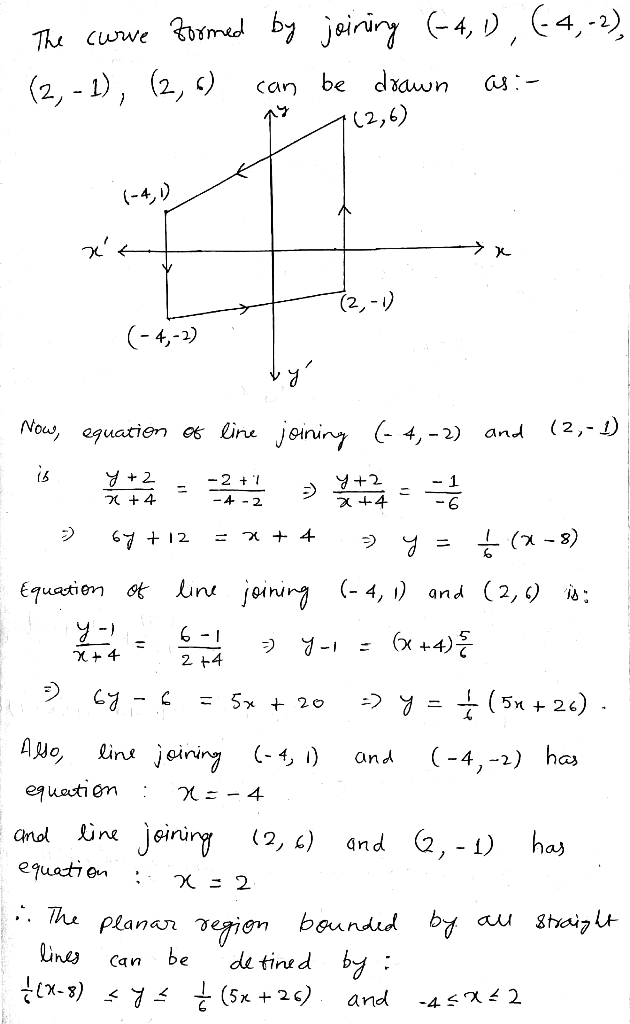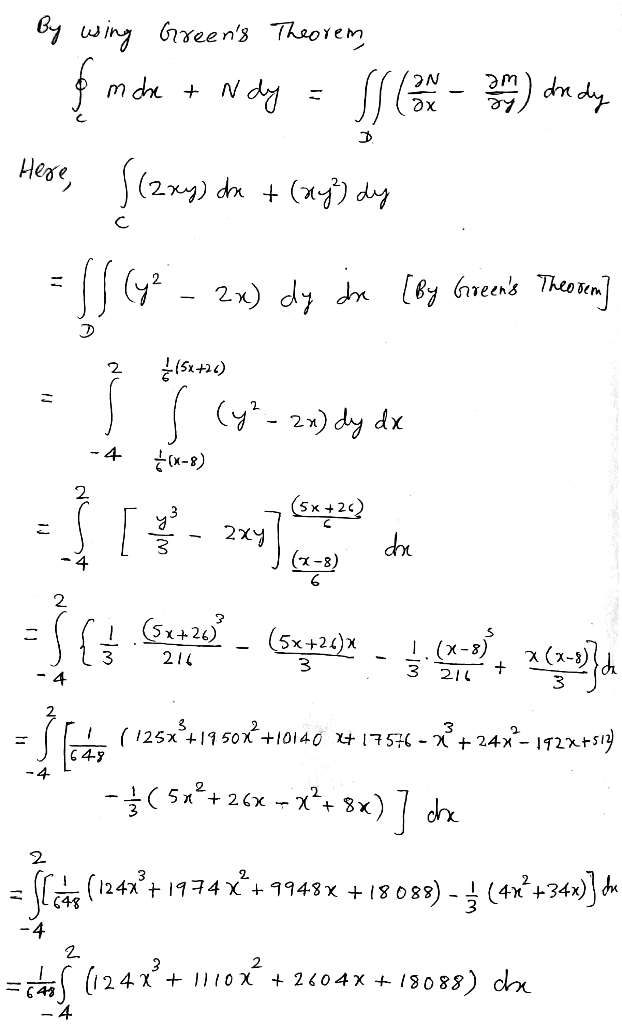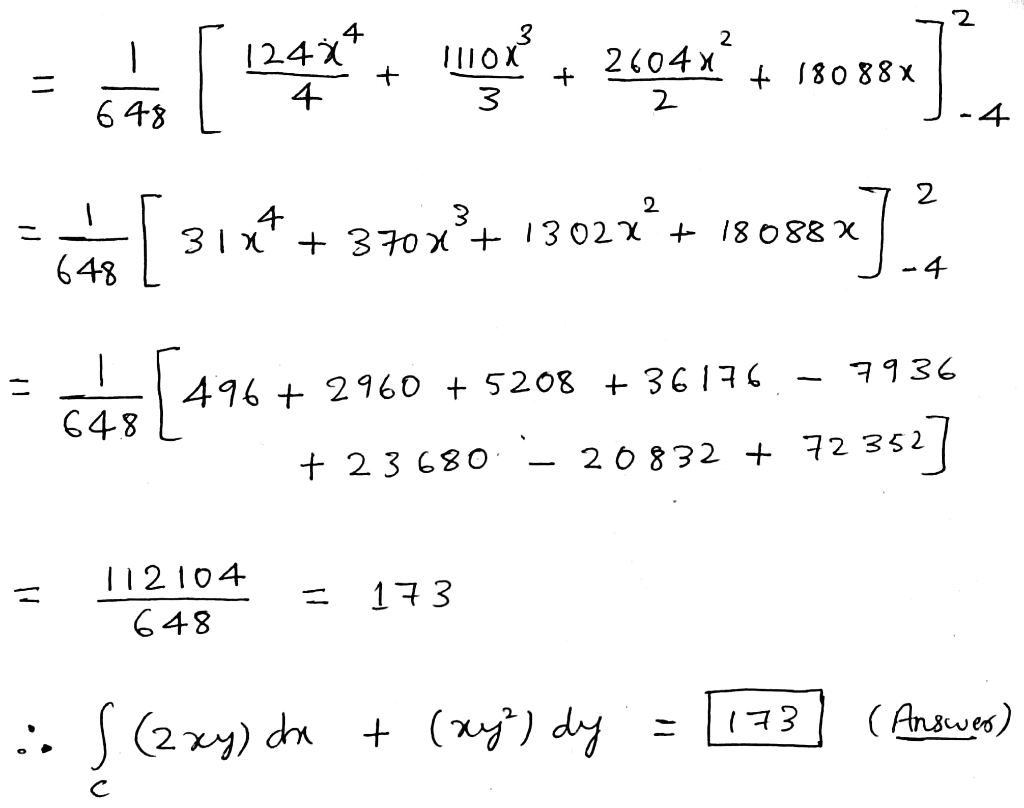##### Add Answer of: Let C be the closed, piecewise smooth curve formed by traveling in straight lines between the points (−4, 1), (−4, −2),...
Similar Homework Help Questions
• ### (a) Let S be the area of a bounded and closed region D with boundary дD of a smooth and simple cl...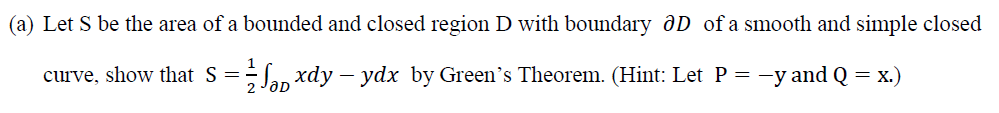(a) Let S be the area of a bounded and closed region D with boundary дD of a smooth and simple closed curve, show that S Jlxy -ydx by Green's Theorem. (Hint: Let P--yandQ x) (b) Let D = {(x,y) 1} be an ellipse, compute the area of D a2 b2 (c) Let L be the upper half from point A(a, 0) to point B(-a, 0) along the elliptical boundary, compute line integral I(e* siny - my)dx + (e* cos...

• ### (b) Let C be the closed curve formed by intersecting the cylinder x2 +y= 1 with the plane x z= 2. Let the tangent to th...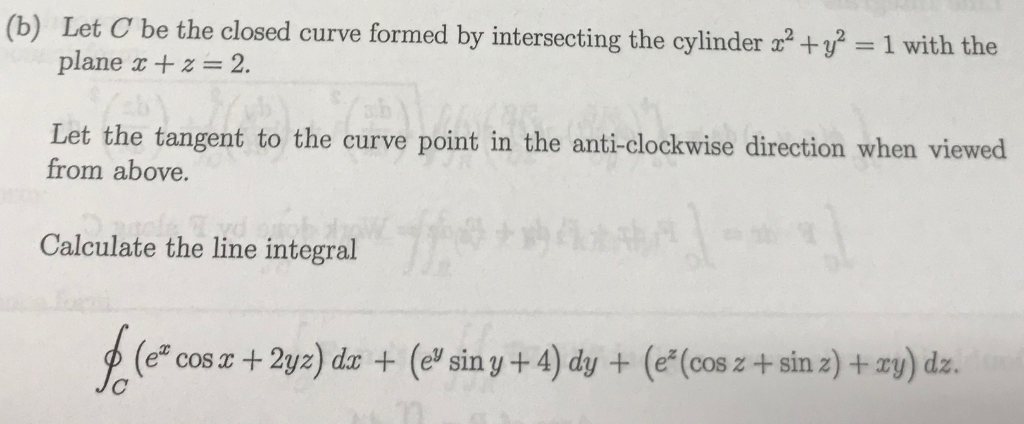(b) Let C be the closed curve formed by intersecting the cylinder x2 +y= 1 with the plane x z= 2. Let the tangent to the curve from above. point in the anti-clockwise direction when viewed Calculate the line integral (e (e sin y+ 4) dy+(e(cos z+ sin z)+ay) dz. cos x2yz) dx + (b) Let C be the closed curve formed by intersecting the cylinder x2 +y= 1 with the plane x z= 2. Let the tangent to the...

• ### (a3, y3,4z3). Let Si be the disk in the 12. Consider the vector field in space...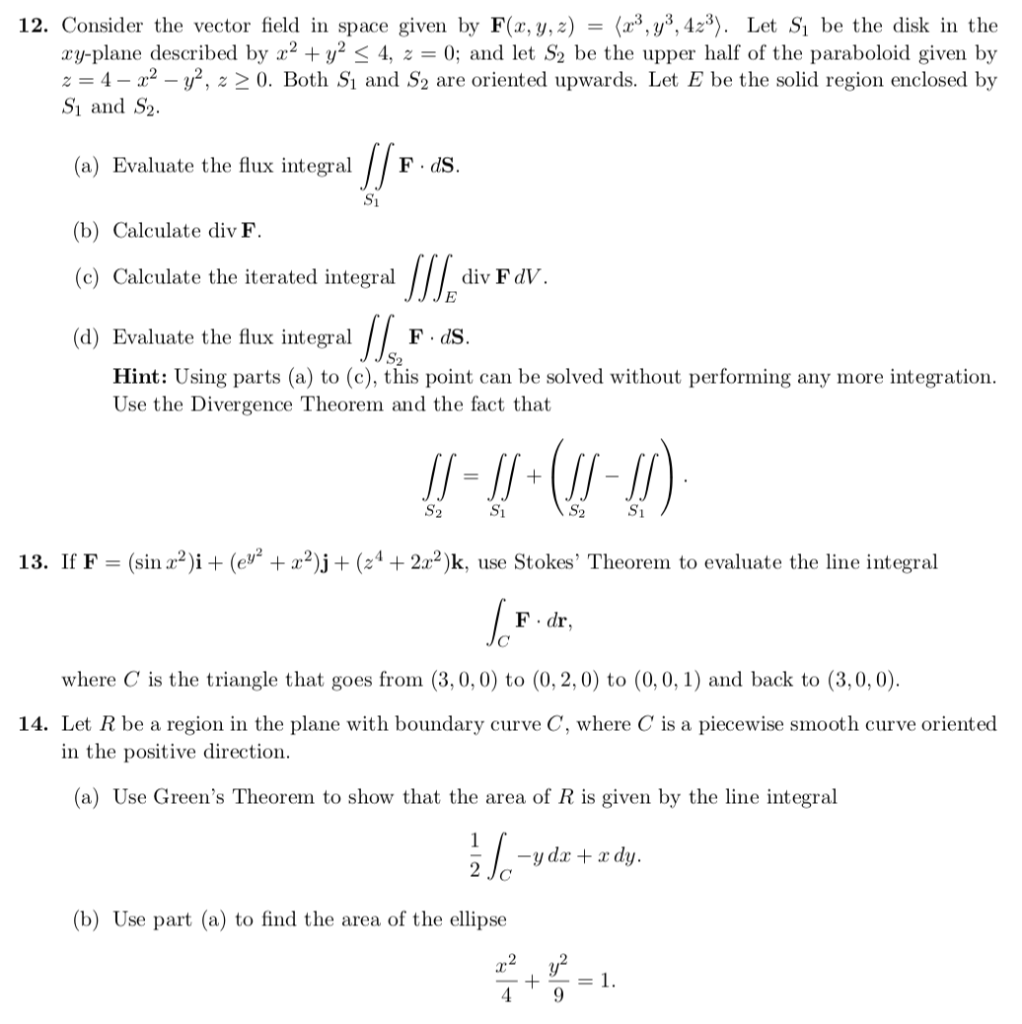(a3, y3,4z3). Let Si be the disk in the 12. Consider the vector field in space given by F(x, y, z) xy-plan described by x2 + y2 < 4, z = 0; and let S2 be the upper half of the paraboloid given by z 4 y2, z 2 0. Both Si and S2 are oriented upwards. Let E be the solid region enclosed by S1 and S2 (a) Evaluate the flux integral FdS (b) Calculate div F div F...

• ### Fundamental Theorem of Line IntegralsLet C be a smooth curve in the xy-plane beginning at (-2,3) andending at (0,-1).Use the Fundamental Theorem of LineIntegrals to Evaluate: + (2xy + 2)dyAnd it has a C at the lower end of the integral sign.

• ### 2. (3 pts.) Let C denote the unit circle, oriented clockwise. Evaluate the line integral ydx dy in two different ways: first by parameterizing the curve and using the definition of line integral;...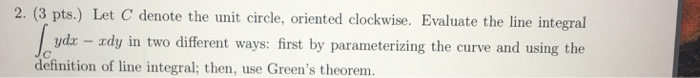2. (3 pts.) Let C denote the unit circle, oriented clockwise. Evaluate the line integral ydx dy in two different ways: first by parameterizing the curve and using the definition of line integral; then, use Green's theorem. 2. (3 pts.) Let C denote the unit circle, oriented clockwise. Evaluate the line integral ydx dy in two different ways: first by parameterizing the curve and using the definition of line integral; then, use Green's theorem.

• ### Problem 13. (1 point) Use Green's theorem to evaluate [4(-y +y)dx +4(x + 2xy)] dy. where C is the rectangle with vertices (0, 0), (5, 0) (5, 2) and (0, 2). A.1-20 B. I 160 DEI 40 Problem...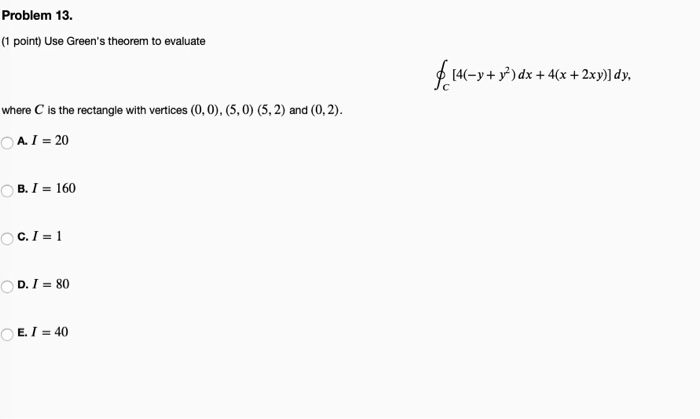Problem 13. (1 point) Use Green's theorem to evaluate [4(-y +y)dx +4(x + 2xy)] dy. where C is the rectangle with vertices (0, 0), (5, 0) (5, 2) and (0, 2). A.1-20 B. I 160 DEI 40 Problem 13. (1 point) Use Green's theorem to evaluate [4(-y +y)dx +4(x + 2xy)] dy. where C is the rectangle with vertices (0, 0), (5, 0) (5, 2) and (0, 2). A.1-20 B. I 160 DEI 40

• ### Evaluate ?(sub C)(x+y)dx-ydy, where C is the closed curve formed by moving along y= 2x² from...

Evaluate ?(sub C)(x+y)dx-ydy, where C is the closed curve formed by moving alongy= 2x² from (0,0) to (2, 8), and then back along the line segment from (2, 8) to (0, 0), using:(a) Line Integrals(b) Green’s Theorem

• ### QB(27pts)(a). Evaluate the circulation ofF(xy)-<x,y+x> on the curve r(t)=<2cost, 2sinp, foross2n (b) Evaluate J F.dr, where C is a piecewise smooth path from (1,0) to (2,1) and F- (e&#39...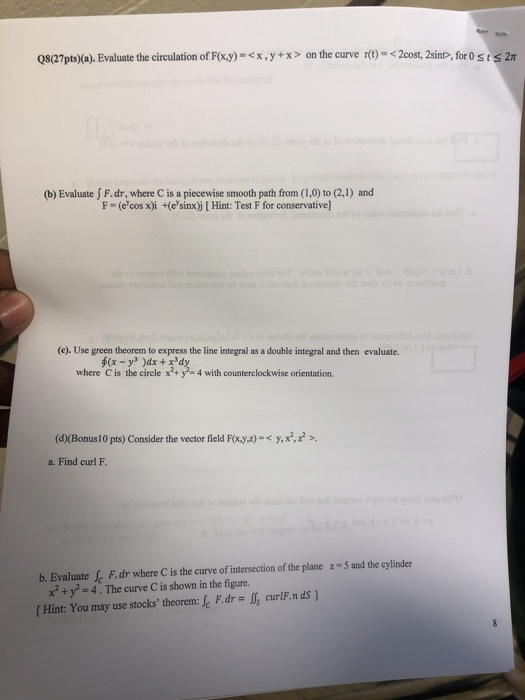QB(27pts)(a). Evaluate the circulation ofF(xy)-<x,y+x> on the curve r(t)=<2cost, 2sinp, foross2n (b) Evaluate J F.dr, where C is a piecewise smooth path from (1,0) to (2,1) and F- (e'cos x)i +(e'sinx)j [Hint: Test F for conservative (c). Use green theorem to express the line integral as a double integral and then evaluate. where C is the circle x+y-4 with counterclockwise orientation. (d(Bonus10 pts) Consider the vector field Foxyz) a. Find curl F y, ,z> F.dr where C is the curve...

• ### there is first question E then there is the question of the value of the line integral ,then quwstion A, then question 1, and the last two pictures are one question Question E...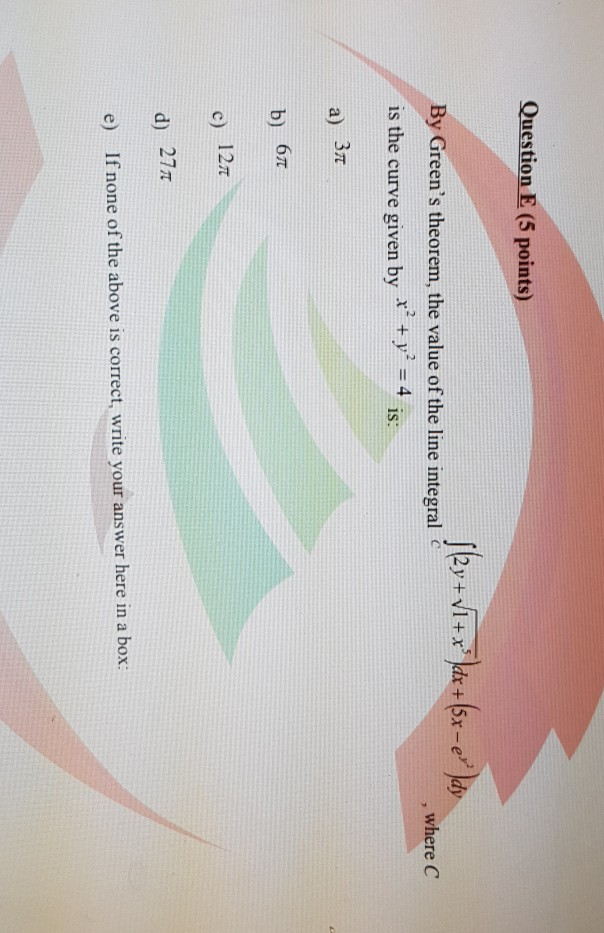there is first question E then there is the question of the value of the line integral ,then quwstion A, then question 1, and the last two pictures are one question Question E (5 points) By Green's theorem, the value of the line integral y 4 is: , where C is the curve given by a) 3 c) 12t d) 27T e) If none of the above is correct, write your answer here in a box rover the line segment...

• ### 3) (11 points) Consider the vector field Use the Fundamental Theorem of lLine Integrals to find the work done by F along any curve from 41. 1Le) to B(2. el) 4) (10 points) Consider the vec...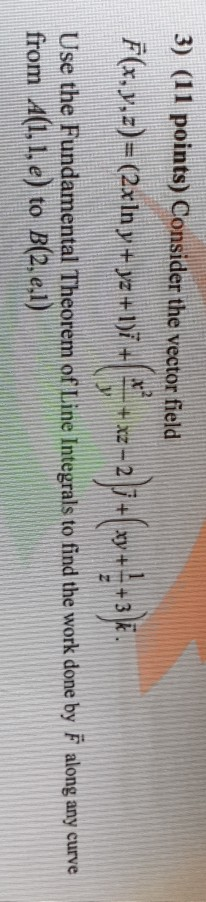3) (11 points) Consider the vector field Use the Fundamental Theorem of lLine Integrals to find the work done by F along any curve from 41. 1Le) to B(2. el) 4) (10 points) Consider the vector field F(x.y)-(r-yi+r+y)j and the circle C: r y-9. Verify Green's Theorem by calculating the outward flux of F across C (12 points) Find the absolute extreme values of the function .-2-4--3 on the closed triangular region in the xy-plane bounded by the lines x...

Need Online Homework Help?